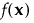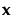Caution: For a design with nominal factors, the matrix in the Model Matrix script saved by the Save X Matrix option is not the coding matrix used in fitting the linear model. You can obtain the coding matrix used for fitting the model by selecting the option Save Columns > Save Coding Table in the Fit Model report that you obtain when you run the Model script.
Note: You can set a preference to always save the matrix script. Select File > Preferences > Platforms > DOE. Check Save X Matrix.
The model matrix describes the design for the experiment. The model matrix has a row for each run and a column for each term of the model specified in the Model outline. For each run, the corresponding row of the model matrix contains the coded values of the model terms:
 • Continuous terms are coded to range from -1 to 1.
 • Nominal terms are coded by applying the Gram-Schmidt orthogonalization procedure to the coding for nominal effects that is used in fitting linear models.
The moments matrix is dependent upon the model effects but is independent of the design. It is defined as follows:
wheredenotes the model effects corresponding to factor combinations of the vector of factors,, and R denotes the design space. For additional details concerning moments and design matrices, see Goos and Jones (2011, pp 88-90) and Myers et al. (2009). Note that the moments matrix is called a matrix of region moments in Myers et al. (2009, p. 376).
From the Custom Design red triangle menu, select Save X Matrix. After the design and the table are created, in the Custom Design table, the Moments Matrix and Model Matrix scripts, and if the design is a split plot, the V Inverse script, are saved as table properties.
 • Select Edit from the red triangle next to either the Moments Matrix, Model Matrix, or V Inverse script. The script shows the corresponding matrix. You can copy this matrix into scripts that you write.
 • When you run the Moments Matrix script, the log shows the number of rows in the moments matrix, called Moments.
 • When you run the script Model Matrix, the log displays the number of rows in the model matrix, called X.
 • When you run the script V Inverse, the log displays the number of rows in the inverse covariance matrix, called V Inverse.
Tip: To see the log, select View > Log (Window > Log on the Macintosh).
 1 Select DOE > Custom Design.
 2 Add 3 continuous factors and click Continue.
 3 Click Interactions > 2nd.
 4 From the Custom Design red triangle menu, select Save X Matrix.
 5 Using the Default Number of Runs (12), click Make Design and then Make Table.
 6
Moments Matrix Script
 7 If it is not already open, select View > Log (Window > Log on the Macintosh).
 8 In the Table panel, click the green triangle next to the Moments Matrix script.
 9
 10 Click Run.
 11 To view the Model Matrix as a data table, add these lines to the script:
`dt = New Table( "Model Matrix" );`
`dt << Set Matrix( X );`
 12 Click Run.

Help created on 9/19/2017